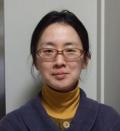### OISHI-TOMIYASU, Ryoko

Professor

My research area is number theory including the theory and application of lattices and quadratic forms.
Around 2011-2014, I developed computational methods that can be used for lattice determination from experimental data, and implemented them into the software CONOGRAPH with help of researchers of KEK. Since the CONOGRAPH method could be applied to different types of experimental data, I have been working in the field of mathematical crystallography since then, collaborating with scientists. Through the applied research, I’m always finding various problems related to lattices, discrete point sets and numerical analysis, and how useful number theory, harmonic analysis and optimization methods are to resolve them.

Keywords Applied Algebra / Number Theory, Mathematical Crystallography, Algorithm Division of Intelligent Societal Implementation of Mathematical Computation Homepage